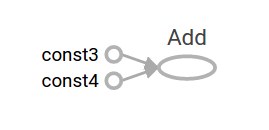## TensorFlow 学习 -- 编程人员指南: 低级别 API 简介 （1）

1449 0

### 快来加入 TensorFlowers 大家庭！

x

• 管理您自己的 TensorFlow 程序 (tf.Graph) 和 TensorFlow 运行时 (tf.Session)，而不是依靠 Estimator 来管理它们。
• 使用 tf.Session 来运行 TensorFlow 指令。
• 在此低级别环境中使用高级别组件（数据集feature_columns）。
• 构建自己的训练循环，而不是使用 Estimator 提供的训练循环。

• 如果您能够直接使用低级别的 TensorFlow 指令，实验和调试都会更直接。
• 您在使用更高级别的 API 时，能够理解其内部工作原理。

• 如何使用 Python 编程。
• 对阵列有所了解。
• 理想情况下，最好对机器学习有一定的了解。

from __future__ import absolute_import
from __future__ import division
from __future__ import print_function

import numpy as np
import tensorflow as tf

TensorFlow 中的核心数据单位是张量。一个张量由一组形成阵列（任意维数）的原始值组成。张量的是它的维数，而它的形状是一个整数元组，指定了阵列每个维度的长度。以下是张量值的一些示例：

3. # a rank 0 tensor; a scalar with shape [],
[1., 2., 3.] # a rank 1 tensor; a vector with shape 
[[1., 2., 3.], [4., 5., 6.]] # a rank 2 tensor; a matrix with shape [2, 3]
[[[1., 2., 3.]], [[7., 8., 9.]]] # a rank 3 tensor with shape [2, 1, 3]
TensorFlow 使用 numpy 阵列来表示张量
TensorFlow Core 演示

• 指令（或“op"）：图的节点。 指令说明的是消耗和生成张量的计算。
• 张量：图的边。它们代表将流经图的值。大多数 TensorFlow 函数会返回 tf.Tensors。

a = tf.constant(3.0, dtype=tf.float32)
b = tf.constant(4.0) # also tf.float32 implicitly
total = a + b
print(a)
print(b)
print(total)

Tensor("Const:0", shape=(), dtype=float32)
Tensor("Const_1:0", shape=(), dtype=float32)

TensorBoard
TensorFlow 提供了一个名为 TensorBoard 的实用程序。TensorBoard 的诸多功能之一是将计算图可视化。您只需要使用几个简单的命令就能轻松完成此操作。

writer = tf.summary.FileWriter('.')

events.out.tfevents.{timestamp}.{hostname}

tensorboard --logdir .sess = tf.Session()
print(sess.run(total))

7.0

print(sess.run({'ab':(a, b), 'total':total}))

{'total': 7.0, 'ab': (3.0, 4.0)}

vec = tf.random_uniform(shape=(3,))
out1 = vec + 1
out2 = vec + 2
print(sess.run(vec))
print(sess.run(vec))
print(sess.run((out1, out2)))

[ 0.52917576  0.64076328  0.68353939]
[ 0.66192627  0.89126778  0.06254101]
(
array([ 1.88408756,  1.87149239,  1.84057522], dtype=float32),
array([ 2.88408756,  2.87149239,  2.84057522], dtype=float32)
)本版积分规则 回帖后跳转到最后一页## 新帖排行